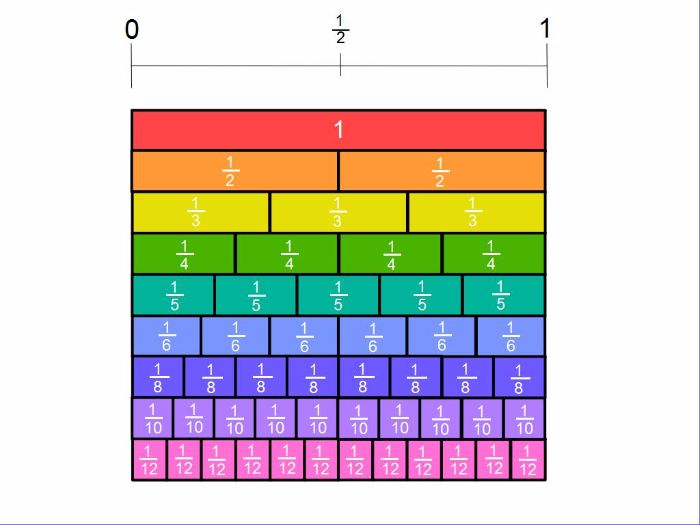This week we are revisiting fractions. We have been using concrete resources to learn about equivalent fractions (fractions that have the same value as each other).  Earlier in the year we drew our own fraction walls. We are revisiting fraction walls when ordering fractions on number lines.Next week we will be working on how to express a number as a fraction. We will also be solving real-life problems based on fractions.

You can use the fraction wall at home to talk about equivalent fractions.

The website below has some great games to play.

http://sheppardsoftware.com/math.htm#fractions

If you want more of a challenge I have also assigned tasks for the girls on Mangahigh, they can use their login code to access.

Useful language when using fractions: fractions, equivalence, equal, the same value, bigger/smaller, amount, numerator, denominator.